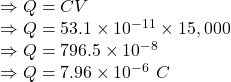Question

8. Consider a capacitor that is made of two large conducting plates that are rectangular in shape (1 cm by 6 cm), aligned parallel to each other, and separated by an air-filled gap of 0.001 cm. This capacitor is included in a circuit where a battery provides 15,000 V of potential difference. When the capacitor is fully charged in this circuit, what is the c

1.Answer: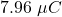Explanation:

Given

The dimension of the plate is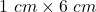The gap between the plate is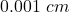Voltage applied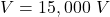The capacitance of the capacitor is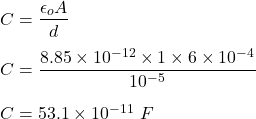Charge acquired by the capacitor